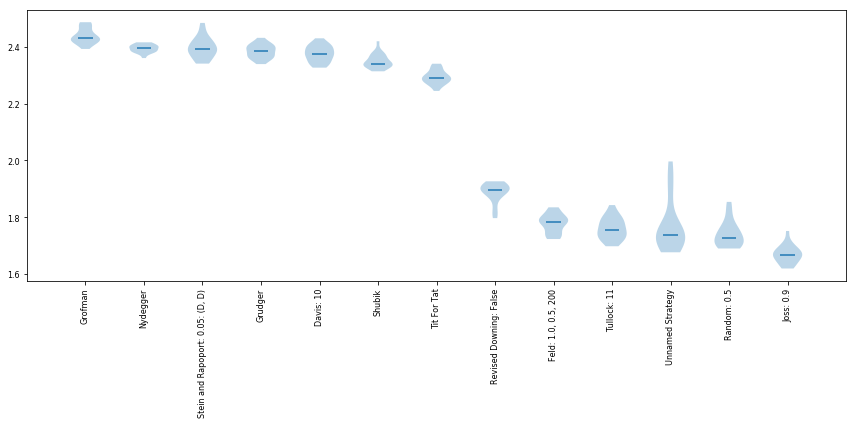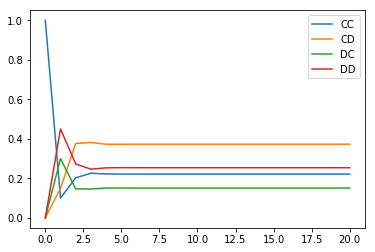# Prisoners Dilemma¶

Video

One big area of repeated games is specifically the Prisoner's Dilemma. We have previously defined this as the following game:

$$A = \begin{pmatrix} 3 & 0\\ 5 & 1 \end{pmatrix}\qquad B = \begin{pmatrix} 3 & 5\\ 0 & 1 \end{pmatrix}$$

The general form is:

$$A = \begin{pmatrix} R & S\\ T & P \end{pmatrix}\qquad B = \begin{pmatrix} R & T\\ S & P \end{pmatrix}$$

with the following constraints:

$$T > R > P > S$$$$2R > T + S$$
• The first constraint ensures that the second action "Defect" dominates the first action "Cooperate".
• The second constraint ensures that a social dilemma arises: the sum of the utilities to both players is best when they both cooperate.

This game is a good model of agent (human, etc) interaction: a player can choose to take a slight loss of utility for the benefit of the other play and themselves.

As a single one shot game there is not much more to say about the Prisoner's dilemma. It becomes fascinating when studied as a repeated game.

## Axelrod's tournaments¶

Video

In 1980, Robert Axelrod (a political scientist) invited submissions to a computer tournament version of an iterated prisoners dilemma. This was described in a 1980 paper titled "Effective Choice in the Prisoner's Dilemma".

### First tournament¶

• 15 strategies submitted.
• Round robin tournament with 200 stages including a 16th player who played uniformly randomly.
• Some very complicated strategies, including for example a strategy that used a $\chi^2$ test to try and identify strategies that were acting randomly. You can read more about this tournament here: http://axelrod.readthedocs.io/en/stable/reference/overview_of_strategies.html#axelrod-s-first-tournament
• The winner (average score) was in fact a very simple strategy: Tit For Tat. This strategy starts by cooperating and then repeats the opponents previous move.

The fact that Tit For Tat won garnered a lot of research (still ongoing) as it showed a mathematical model of how cooperative behaviour can emerge in complex situations (why are we nice to each other?).

There is a Python library (axelrod) with over 200 strategies that can be used to reproduce this work. You can read the documentation for it here: http://axelrod.readthedocs.io.

Note that in version '3.1.0' two of the original strategies are not implemented so here is the best reproduction of Axelrod's tournament:

In :
%matplotlib inline

import axelrod as axl
assert axl.__version__ == '3.6.0'

axl.seed(0)  # Make this reproducible
players = [axl.TitForTat(),
axl.Nydegger(),
axl.Grofman(),
axl.Shubik(),
axl.SteinAndRapoport(),
axl.Grudger(),
axl.Davis(),
axl.RevisedDowning(revised=False),
axl.Feld(),
axl.Joss(),
axl.Tullock(),
axl.UnnamedStrategy(),
axl.Random()]
tournament = axl.Tournament(players, turns=200, repetitions=20)
results = tournament.play()
plot = axl.Plot(results)
plot.boxplot();

Playing matches: 100%|██████████| 91/91 [00:04<00:00, 18.43it/s]
Analysing: 100%|██████████| 1820/1820 [00:04<00:00, 394.14it/s]
Finishing: 100%|██████████| 39/39 [00:00<00:00, 903.60it/s]We see that Tit For Tat does in fact not win this tournament. This highlights that there is no such thing as a best strategy but a best strategy for a particular environment.

Here is some of the source code for the strategies:

In :
axl.TitForTat.strategy??


Tit For Tat:

Signature: axl.TitForTat.strategy(self, opponent:axelrod.player.Player) -> str
Source:
def strategy(self, opponent: Player) -> Action:
"""This is the actual strategy"""
# First move
if not self.history:
return C
# React to the opponent's last move
if opponent.history[-1] == D:
return D
return C
File:      ~/anaconda3/envs/gt/lib/python3.6/site-packages/axelrod/strategies/titfortat.py
Type:      function

In :
axl.Grudger.strategy??

Signature: axl.Grudger.strategy(opponent:axelrod.player.Player) -> str
Source:
@staticmethod
def strategy(opponent: Player) -> Action:
"""Begins by playing C, then plays D for the remaining rounds if the
opponent ever plays D."""
if opponent.defections:
return D
return C
File:      ~/anaconda3/envs/gt/lib/python3.6/site-packages/axelrod/strategies/grudger.py
Type:      function


## Reactive strategies¶

Video

In 1989 a particular family of strategies was introduced by Martin Nowak. These strategies are defined by two parameters: $(p_1, p_1)$ where:

• $p_1$ is the probability of cooperating after an opponent cooperates;
• $p_2$ is the probability of cooperating after an opponent defects.

## Markov chain representation of a Match between two reactive strategies¶

Consider two reactive players:

$$p=(p_1, p_2) \qquad q=(q_1, q_2)$$

If we consider the order of possible states of a match to be:

$$S=\{CC, CD, DC, DD\}$$

then we can summarise a game with the following matrix:

$$M = \begin{pmatrix} p_1q_1 & p_1(1-q_1) & (1-p_1)q_1 & (1-p_1)(1-q_1) \\ p_2q_1 & p_2(1-q_1) & (1-p_2)q_1 & (1-p_2)(1-q_1) \\ p_1q_2 & p_1(1-q_2) & (1-p_1)q_2 & (1-p_1)(1-q_2) \\ p_2q_2 & p_2(1-q_2) & (1-p_2)q_2 & (1-p_2)(1-q_2) \\ \end{pmatrix}$$

The matrix $M$ corresponds to a Markov chain. Given a probability vector $\pi$ representing the probability of being in a given state of $S$: the probabilities of being in the a given step in the next round are given by:

$$\pi M$$

If we consider:

$$p=(1 / 4, 4 / 5) \qquad q=(2 / 5, 1 / 3)$$

below is some code that calculates the probabilities over 20 turns starting with both strategies cooperating:

In :
import numpy as np
import matplotlib.pyplot as plt
v_1 = np.array([1 / 4, 4 / 5])
v_2 = np.array([2 / 5, 1 / 3])

M = np.array([[v_1 * v_2, v_1 * (1 - v_2), (1 - v_1) * v_2,  (1 - v_1) * (1 - v_2)],
[v_1 * v_2, v_1 * (1 - v_2), (1 - v_1) * v_2,  (1 - v_1) * (1 - v_2)],
[v_1 * v_2, v_1 * (1 - v_2), (1 - v_1) * v_2,  (1 - v_1) * (1 - v_2)],
[v_1 * v_2, v_1 * (1 - v_2), (1 - v_1) * v_2,  (1 - v_1) * (1 - v_2)]])

pis = [np.array([1, 0, 0, 0])]
number_of_turns = 20
for _ in range(number_of_turns):
pis.append(np.dot(pis[-1], M))

labels = ["CC", "CD", "DC", "DD"]
for state, label in zip(zip(*pis), labels):
plt.plot(state, label=label)
plt.legend();We see that over time these probabilities no longer change: this is referred to as steady state. A probability vector $\pi$ at steady state is a solution to the following matrix equation:

$$\pi M=\pi$$

## Theorem: steady state probabilities for match between reactive players¶

The steady state of a match between (non deterministic) reactive players is given by:

$$\pi=(s_1s_2, s_1(1-s_2), (1-s_1)s_2, (1-s_1)(1-s_2))$$

where:

$$s_1 = \frac{q_2r_1+p_2}{1-r_1r_2}\qquad s_2 = \frac{p_2r_2+q_2}{1-r_1r_2}$$

for:

$$r_1=p_1-p_2\qquad r_2=q_1-q_2$$

### Proof¶

The proof follow (after some heavy algebra) by carrying out the following multiplication:

$$\pi M = (s_1s_2, s1(1-s_2), (1-s_1)s_2, (1-s_1)(1-s_2)) \begin{pmatrix} p_1q_1 & p_1(1-q_1) & (1-p_1)q_1 & (1-p_1)(1-q_1) \\ p_2q_1 & p_2(1-q_1) & (1-p_2)q_1 & (1-p_2)(1-q_1) \\ p_1q_2 & p_1(1-q_2) & (1-p_1)q_2 & (1-p_1)(1-q_2) \\ p_2q_2 & p_2(1-q_2) & (1-p_2)q_2 & (1-p_2)(1-q_2) \\ \end{pmatrix}$$

Using this we can obtain the expected utility of the first player:

$$s_1s_2\times R + s1(1-s_2) \times S + (1-s_1)s_2 \times T + (1-s_1)(1-s_2)\times P$$

The second player:

$$s_1s_2\times R + s1(1-s_2) \times T + (1-s_1)s_2 \times S + (1-s_1)(1-s_2)\times P$$
In :
def theoretic_steady_state(p, q):
r_1 = p - p
r_2 = q - q
s_1 = (q * r_1 + p) / (1 - r_1 * r_2)
s_2 = (p * r_2 + q) / (1 - r_1 * r_2)
return np.array([s_1 * s_2, s_1 * (1 - s_2), (1 - s_1) * s_2, (1 - s_1) * (1 - s_2)])

def theoretic_utility(p, q, rstp=np.array([3, 0, 5, 1])):
pi = theoretic_steady_state(p, q)
return np.dot(pi, rstp)

In :
theoretic_utility(v_1, v_2), theoretic_utility(v_2, v_1)

Out:
(1.6752308185399241, 2.7845555773823678)

We can confirm this using the Axelrod library:

In :
player_1 = axl.ReactivePlayer(probabilities=v_1)
player_2 = axl.ReactivePlayer(probabilities=v_2)
axl.seed(0)
match = axl.Match(players=(player_1, player_2), turns=5000)
interactions = match.play()
match.final_score_per_turn()

Out:
(1.654, 2.799)

## Numerous variants of this tournament have since been carried out:¶

Previous

Next

Source code: @drvinceknight Powered by: Python Mathjax Github pages Skeleton css